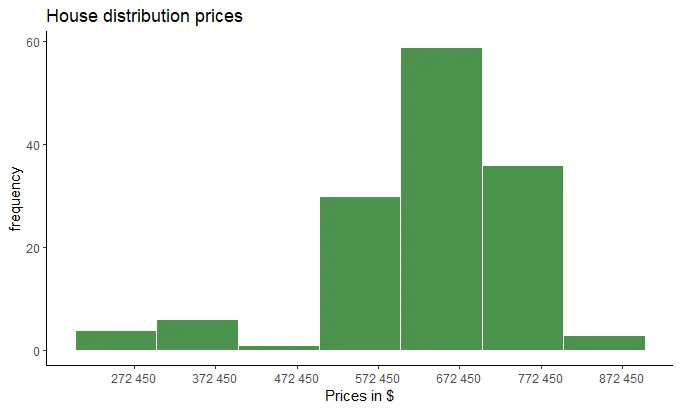18 Jun 2022

Posted on:

12 Jun 2022

0

# Histogram configuration x axis

Hi there, i have been practising histogram on my own with some excerciss from statitics course. So! i want to manipulate better the intervals in order to have my histograms between the intervals i want to show.

In the histogram attached, you can see that the boundaries of my x axix does not start either at the begining of any of my bars or at the end

I almost never ask in the Q&A but this time i just ran out of options. I hope the question is clear. thanks in advance.

``````#THIS IS THE CODE USED FOR THE CHART
point <- format_format(big.mark = " ", decimal.mark = ",", scientific = FALSE)

House.hist <- ggplot(House, aes(x = Price))

House.hist +
geom_histogram(binwidth = 100000, boundary = 0,
color = "white", fill = "darkgreen", alpha = 0.7) +
theme_classic() +
scale_x_continuous(labels = point,
breaks = seq(272450, 972450, 100000)) +
labs(title = "House distribution prices",
x = "Prices in \$",
y = "frequency")
``````Instructor
Posted on:

16 Jun 2022

0

Hi Carlos,
thanks for reaching out! I was unable to reproduce your code, for some reason the format_format() function gives me an error, could you please include the libraries you used for the code, ideally if you know where format_format comes from.